## The Measurement of Angles

In the study of geometry, angle measures are often thought of as a degree of separation between two rays with a common endpoint, or possibly associated with the size of a given sector of a circle. Both of these interpretations implicitly place restrictions on what measures angles are allowed to have. For example, in these contexts, angles never have negative measures. After all, what would it mean to have less than zero separation between two rays with a common endpoint? Likewise, angles measures can never exceed $360^{\circ}$. How after all, could one have a sector of a circle that exceeded the circle itself? The following discussion provides a re-interpretation angles as rotations, which allows us to generalize angles so that their measures may take on any real value, regardless of its sign or magnitude.

An angle is formed by two half-lines having a common endpoint called a vertex. One half-line is designated the initial side and the other the terminal side. We may consider the angle as having been formed by a rotation from the initial to the terminal side.

When we place the angle in the Cartesian coordinate plane with its vertex at the origin and initial side coinciding with the positive $x$-axis, we say that the angle is in standard position. In future discussions, we will consider angles in their standard positions.

### Degree Measure of Angles

The angle formed by one complete counterclockwise rotation is assigned degree measure $360^{\circ}$. Other angles are then assigned degree measures proportionally.

So for example, the angle formed by two complete, counterclockwise rotations measures $2 \cdot 360^{\circ} = 720^{\circ}$, while the angle formed by a quarter of a counter-clockwise rotation measures only $\frac{1}{4} \cdot 360^{\circ} = 90^{\circ}$.

The sign of the measure indicates the direction of rotation, and is given by:

 $(+)$ if rotation is counterclockwise $(-)$ if rotation is clockwise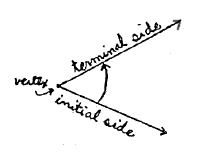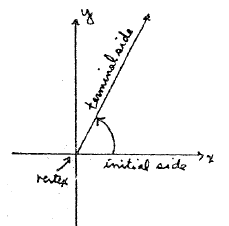Angle in Standard Position

Examples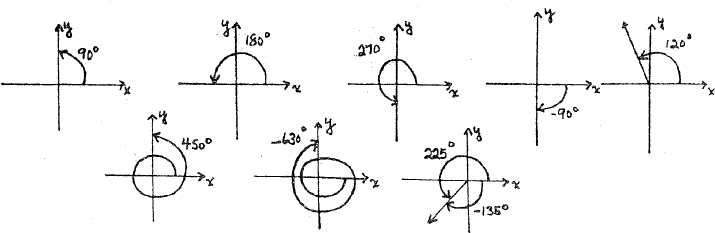Practice

### Coterminal Angles

When two angles have the same initial and terminal sides, they are said to be coterminal angles.

Examples

• Angles of $960^{\circ}$ and $240^{\circ}$ are coterminal angles.
• Angles of $-675^{\circ}$, $-315^{\circ}$, and $45^{\circ}$ are coterminal angles.
In general, the angle $\theta$ and the angle $\theta \pm n(360^{\circ})$, for $n=1,2,3,\ldots$ are coterminal.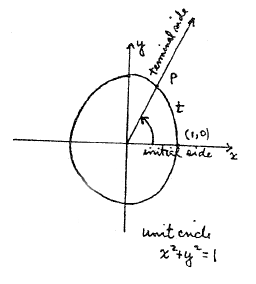The radian measure of an angle is based on the length of an arc on the unit circle given by $$\{(x,y) : x^2 + y^2 = 1\}$$ We measure the angle by the distance $t$ traversed along the circumference of the unit circle as the angle is generated by a rotation from the initial side to the terminal side in standard position.

The sign conventions are as they were before:

• $(+)$ if rotation is counterclockwise
• $(-)$ if rotation is clockwise

The radian measure of the angle formed by one complete counter-clockwise rotation equals the circumference of the unit circle, which is $2 \pi$. (Recall, $C=2\pi r$ and $r=1$ in a unit circle).

Other examples are given below: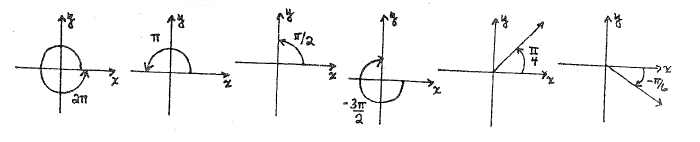### Converting Between Radians and Degrees

Noting that $\pi \textrm{ radians } = 180^{\circ}$, or equivalently $\displaystyle{1^{\circ} = \frac{\pi}{180} \textrm{ radians}}$, we can convert the degree measure of any angle to radians and the radian measure of any angle to degrees.

Practice

• $\displaystyle{20^{\circ} = 20 \left( \frac{\pi}{180} \right) \textrm{ radians } = \frac{\pi}{9} \textrm{ radians}}$

• $\displaystyle{30^{\circ} = 30 \left( \frac{\pi}{180} \right) \textrm{ radians } = \frac{\pi}{6} \textrm{ radians}}$

• $\displaystyle{-60^{\circ} = -60 \left( \frac{\pi}{180} \right) \textrm{ radians } = -\frac{\pi}{3} \textrm{ radians}}$

• Each case is treated in the same way. Since $\pi \textrm{ radians } = 180^{\circ}$, we multiply angle measures in radians by $180^{\circ} \, / \, \pi$ to convert them to angle measures in degrees:

• $\displaystyle{\frac{7\pi}{6} \textrm{ radians } = \frac{7\pi}{6} \cdot \left( \frac{180^{\circ}}{\pi} \right) = 210^{\circ}}$

• $\displaystyle{-\frac{\pi}{12} \textrm{ radians } = -\frac{\pi}{12} \cdot \left( \frac{180^{\circ}}{\pi} \right) = -15^{\circ}}$

• $\displaystyle{0.76 \textrm{ radians } = 0.76 \cdot \left( \frac{180^{\circ}}{\pi} \right) = \left( \frac{136.8}{\pi} \right)^{\circ} \approx 48.54^{\circ}}$